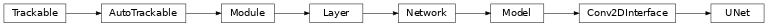# UNet¶

Inheritance Diagramclass ashpy.models.convolutional.unet.UNet(input_res, min_res, kernel_size, initial_filters, filters_cap, channels, use_dropout_encoder=True, use_dropout_decoder=True, dropout_prob=0.3, encoder_non_linearity=<class 'tensorflow.python.keras.layers.advanced_activations.LeakyReLU'>, decoder_non_linearity=<class 'tensorflow.python.keras.layers.advanced_activations.ReLU'>, normalization_layer=<class 'ashpy.layers.instance_normalization.InstanceNormalization'>, last_activation=<function tanh>, use_attention=False)[source]

UNet Architecture.

Architecture similar to the one found in “Image-to-Image Translation with Conditional Adversarial Nets” .

Originally proposed in “U-Net: Convolutional Networks for Biomedical Image Segmentation” .

Examples

• Direct Usage:

x = tf.ones((1, 512, 512, 3))
u_net = UNet(input_res = 512,
min_res=4,
kernel_size=4,
initial_filters=64,
filters_cap=512,
channels=3)
y = u_net(x)
print(y.shape)
print(len(u_net.trainable_variables)>0)

(1, 512, 512, 3)
True

  Image-to-Image Translation with Conditional Adversarial Nets - https://arxiv.org/abs/1611.07004
  U-Net: Convolutional Networks for Biomedical Image Segmentation - https://arxiv.org/abs/1505.04597

Methods

 __init__(input_res, min_res, kernel_size, …) Initialize the UNet. call(inputs[, training]) Forward pass of the UNet model. get_decoder_block(filters[, use_bn, …]) Return a block to be used in the decoder part of the UNET. get_encoder_block(filters[, use_bn, …]) Return a block to be used in the encoder part of the UNET.

Attributes

 activity_regularizer Optional regularizer function for the output of this layer. dtype dynamic inbound_nodes Deprecated, do NOT use! Only for compatibility with external Keras. input Retrieves the input tensor(s) of a layer. input_mask Retrieves the input mask tensor(s) of a layer. input_shape Retrieves the input shape(s) of a layer. input_spec Gets the network’s input specs. layers losses Losses which are associated with this Layer. metrics Returns the model’s metrics added using compile, add_metric APIs. metrics_names Returns the model’s display labels for all outputs. name Returns the name of this module as passed or determined in the ctor. name_scope Returns a tf.name_scope instance for this class. non_trainable_variables non_trainable_weights outbound_nodes Deprecated, do NOT use! Only for compatibility with external Keras. output Retrieves the output tensor(s) of a layer. output_mask Retrieves the output mask tensor(s) of a layer. output_shape Retrieves the output shape(s) of a layer. run_eagerly Settable attribute indicating whether the model should run eagerly. sample_weights state_updates Returns the updates from all layers that are stateful. stateful submodules Sequence of all sub-modules. trainable trainable_variables Sequence of variables owned by this module and it’s submodules. trainable_weights updates variables Returns the list of all layer variables/weights. weights Returns the list of all layer variables/weights.
__init__(input_res, min_res, kernel_size, initial_filters, filters_cap, channels, use_dropout_encoder=True, use_dropout_decoder=True, dropout_prob=0.3, encoder_non_linearity=<class 'tensorflow.python.keras.layers.advanced_activations.LeakyReLU'>, decoder_non_linearity=<class 'tensorflow.python.keras.layers.advanced_activations.ReLU'>, normalization_layer=<class 'ashpy.layers.instance_normalization.InstanceNormalization'>, last_activation=<function tanh>, use_attention=False)[source]

Initialize the UNet.

Parameters: input_res (int) – input resolution. min_res (int) – minimum resolution reached after decode. kernel_size (int) – kernel size used in the network. initial_filters (int) – number of filter of the initial convolution. filters_cap (int) – maximum number of filters. channels (int) – number of output channels. use_dropout_encoder (bool) – whether to use dropout in the encoder module. use_dropout_decoder (bool) – whether to use dropout in the decoder module. dropout_prob (float) – probability of dropout. encoder_non_linearity (Type[Layer]) – non linearity of encoder. decoder_non_linearity (Type[Layer]) – non linearity of decoder. last_activation () – last activation function, tanh or softmax (for semantic images). use_attention (bool) – whether to use attention.
call(inputs, training=False)[source]

Forward pass of the UNet model.

get_decoder_block(filters, use_bn=True, use_dropout=False, use_attention=False)[source]

Return a block to be used in the decoder part of the UNET.

Parameters: filters – number of filters use_bn – whether to use batch normalization use_dropout – whether to use dropout use_attention – whether to use attention A block to be used in the decoder part
get_encoder_block(filters, use_bn=True, use_attention=False)[source]

Return a block to be used in the encoder part of the UNET.

Parameters: filters – number of filters. use_bn – whether to use batch normalization. use_attention – whether to use attention. A block to be used in the encoder part.